# Covering

(diff) ← Older revision | Latest revision (diff) | Newer revision → (diff)

A mappingof a spaceonto a spacesuch that each point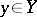has a neighbourhood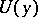the pre-image of which underis a union of open subsets that are mapped homeomorphically ontoby. Equivalently:is a locally trivial fibre bundle with discrete fibre.

Coverings are usually considered on the assumption thatandare connected; it is also usually assumed thatis locally connected and locally simply-connected. Under these assumptions one can establish a relationship between the fundamental groups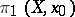and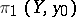: If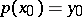, then the induced homomorphism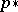mapsisomorphically onto a subgroup ofand, by varying the pointin, one obtains exactly all subgroups in the corresponding class of conjugate subgroups. If this class consists of a single subgroup(i.e. ifis a normal divisor), the covering is said to be regular. In that case one obtains a free action of the group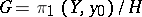on, withplaying the role of the quotient mapping onto the orbit space. This action is generated by lifting loops: If one associates with a loop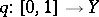,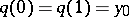, the unique path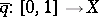such that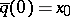and, then the point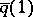will depend only on the class of the loop inand on. Thus, each element ofcorresponds to a permutation of points in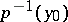. This permutation has no fixed points if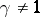, and it depends continuously on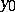. One obtains a homeomorphism of.

In the general case this construction defines only a permutation in, i.e. there is an action ofon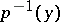, known as the monodromy of the covering. A special case of a regular covering is a universal covering, for which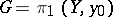. In general, given any subgroup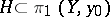, one can construct a unique coveringfor which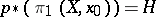. The points ofare the classes of paths,: Two paths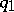andare identified if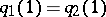and if the loop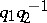lies in an element of. The pointfor the paths of one class is taken as the image of this class; this defines. The topology inis uniquely determined by the condition thatbe a covering; it is here that the local simple-connectedness ofis essential. For any mappingof an arcwise-connected space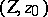into, its lifting into a mapping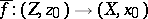exists if and only if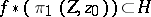. A partial order relation can be defined on the coverings of(a covering of a covering is a covering); this relation is dual to the inclusion of subgroups in. In particular, the universal covering is the unique maximal element.

Examples. The parametrization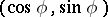of the circle defines a covering of the circle by the real line,, often described in the complex form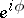and called the exponential covering. Similarly, the torus is covered by the plane. Identification of antipodal points on a sphere yields a covering by the sphere of a projective space of corresponding dimension. In general, free actions of discrete groups are a source of regular coverings (over the orbit space); not every such action yields a covering (the orbit space may be non-separable), but finite groups do.